# Field Oriented Control algorithm briefly v2.0.2

Field oriented control algorithm’s main task is to take user defined voltage uq and, by continuously reading the position of the motor rotor a, calculate the appropriate phase voltages ua,ub and uc.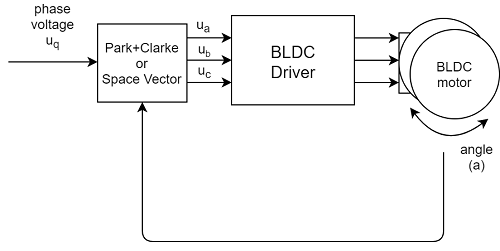FOC algorithm calculates the phase voltages which create the magnetic field in the motor’s rotor which are exactly 90 degrees “behind” the magnetic field of the permanent magnets of the rotor, creating a pushing effect. Here is a very nice animation of what happens inside of the motor when running simplified version of the the FOC called six-step modulation.

Another way to look at why we need 90 degree angle in between rotor and stator fields if by remembering the equation of the electric force generated by the wire passing through the magnetic field:

F = B*I*L*sin(alpha)


Where B is the strength of the magnetic field, L is the length of the wire, I is the electric current magnitude and the alpha is the angle in between magnetic field B and current I. From this equation we can see that in order to have the maximum force F = B*I*L we need to maintain alpha angle equal to 90 degrees or PI/2 radians.

## How to calculate the appropriate voltages ua,ub and uc

Since the SimpleFOClibrary is intended for education about the FOC algorithm as well for enabling various applications, the two most standard versions of the FOC modulation are implemented in this library.

• Sinusoidal PWM: SinePWM
• Space Vector PWM: SpaceVectorPWM

You can configure them by setting the value of motor.foc_modulation variable:

motor.foc_modulation = FOCModulationType::SinePWM; // default
// or
motor.foc_modulation = FOCModulationType::SpaceVectorPWM;


### Sinusoidal modulation SinePWM

Sinusoidal modulation is based on two transformation equations.Inverse Park transformation:Inverse Clarke transformation:Here is the code of implementation of the Sinusoidal PWM in the SimpleFOClibrary:

// Method using FOC to set Uq to the motor at the optimal angle
void BLDCMotor::setPhaseVoltage(float Uq, float angle_el) {
// Sinusoidal PWM modulation
// Inverse Park + Clarke transformation

// angle normalization in between 0 and 2pi
// only necessary if using _sin and _cos - approximation functions
angle_el = normalizeAngle(angle_el + zero_electric_angle);
// Inverse park transform
Ualpha =  -_sin(angle_el) * Uq;  // -sin(angle) * Uq;
Ubeta =  _cos(angle_el) * Uq;    //  cos(angle) * Uq;

// Inverse Clarke transform
Ua = Ualpha + voltage_power_supply/2;
Ub = -0.5 * Ualpha  + _SQRT3_2 * Ubeta + voltage_power_supply/2;
Uc = -0.5 * Ualpha - _SQRT3_2 * Ubeta + voltage_power_supply/2;

// set the voltages in hardware
setPwm(Ua, Ub, Uc);
}


### Space vector modulation SpaceVectorPWM

Space Vector modulation is based on two steps of calculations: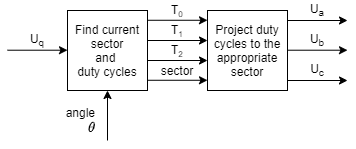In the first step we find the sector s the rotor is currently in. The angle of 360 degrees is divided into 6 equal parts of 60 degrees. So this calculation is very straight forward. Then we calculate the times T0, T1 and T2. T1 and T2 tell us how long should phase 1 and phase 2 be on and T0 tells us how long should we have 0 voltage on the motor.Second step is projecting the T0,1,2 values to the appropriate duty-cycles Ta,b,c which depend directly of the sector the motor is currently in.

SectorTaTbTc
1T1 + T2 + T0/2T2 + T0/2T0/2
2T1 + T0/2T1 + T2 + T0/2T0/2
3T0/2T1 + T2 + T0/2T2 + T0/2
4T0/2T1+ T0/2T1 + T2 + T0/2
5T2 + T0/2T0/2T1 + T2 + T0/2
6T1 + T2 + T0/2T0/2T1 + T0/2

Here is one example of a pwm signal generated using SVM table for parameters: s = 2, T1 = 1/8 = 0.125, T2 = 1/8 = 0.125 and T0 = 1/2 = 0.5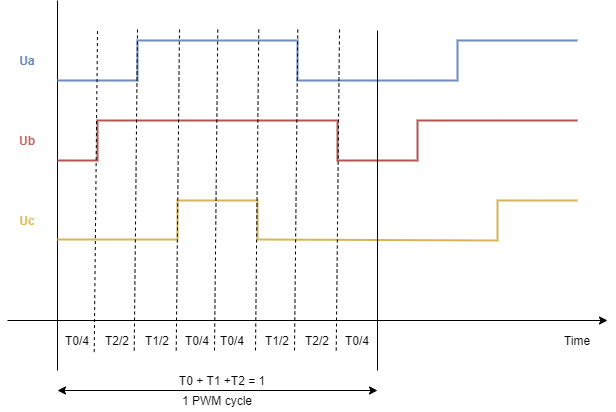Here is the code of the Space Vector PWM implementation in the SimpleFOClibrary:

// Method using FOC to set Uq to the motor at the optimal angle
void BLDCMotor::setPhaseVoltage(float Uq, float angle_el) {
// Nice video explaining the SpaceVectorModulation (SVPWM) algorithm

// if negative voltages change inverse the phase
// angle + 180degrees
if(Uq < 0) angle_el += _PI;
Uq = abs(Uq);

// angle normalisation in between 0 and 2pi
// only necessary if using _sin and _cos - approximation functions
angle_el = normalizeAngle(angle_el + zero_electric_angle + _PI_2);

// find the sector we are in currently
int sector = floor(angle_el / _PI_3) + 1;
// calculate the duty cycles
float T1 = _SQRT3*_sin(sector*_PI_3 - angle_el) * Uq/voltage_power_supply;
float T2 = _SQRT3*_sin(angle_el - (sector-1.0)*_PI_3) * Uq/voltage_power_supply;
// two versions possible
// centered around voltage_power_supply/2
float T0 = 1 - T1 - T2;
// pulled to 0 - better for low power supply voltage
//float T0 = 0;

// calculate the duty cycles(times)
float Ta,Tb,Tc;
switch(sector){
case 1:
Ta = T1 + T2 + T0/2;
Tb = T2 + T0/2;
Tc = T0/2;
break;
case 2:
Ta = T1 +  T0/2;
Tb = T1 + T2 + T0/2;
Tc = T0/2;
break;
case 3:
Ta = T0/2;
Tb = T1 + T2 + T0/2;
Tc = T2 + T0/2;
break;
case 4:
Ta = T0/2;
Tb = T1+ T0/2;
Tc = T1 + T2 + T0/2;
break;
case 5:
Ta = T2 + T0/2;
Tb = T0/2;
Tc = T1 + T2 + T0/2;
break;
case 6:
Ta = T1 + T2 + T0/2;
Tb = T0/2;
Tc = T1 + T0/2;
break;
default:
// possible error state
Ta = 0;
Tb = 0;
Tc = 0;
}

// calculate the phase voltages
Ua = Ta*voltage_power_supply;
Ub = Tb*voltage_power_supply;
Uc = Tc*voltage_power_supply;

// set the voltages in hardware
setPwm(Ua, Ub, Uc);
}


## Which one should I use?

Here are images of the waveforms generated by the Sinusoidal and Space Vector modulation for Uq = 0.5V

SinusoidalSpace Vector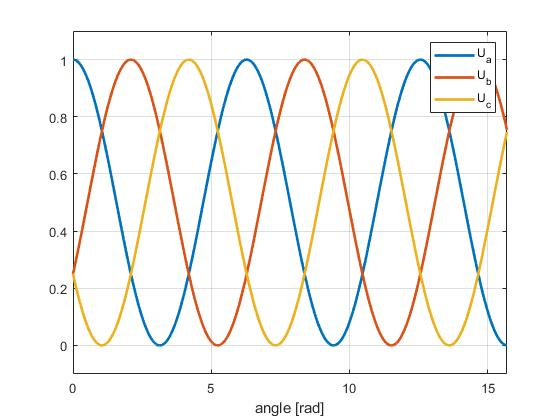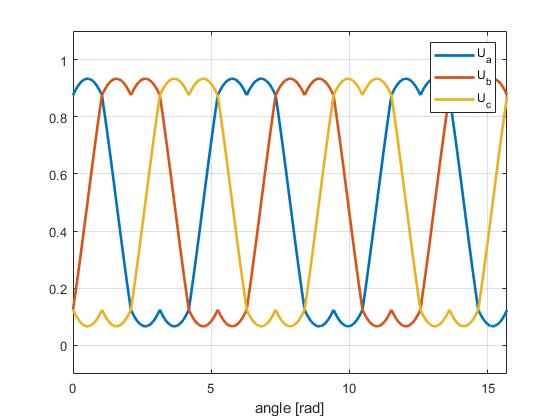There are several key differences in between these two algorithms. But in terms of SimpleFOClibrary all that you need to know is that Space Vector algorithm better uses the maximal voltage range of the power supply. In the tables above, you can see that for Uq = 0.5V the magnitude of sine waves generated by the Sinusoidal modulation is exactly equal to 1, and Space vector modulation is not quiet there yet. The “double sine” wave produced by the space vector has lower magnitude by the factor of 2/sqrt(3) = 1.15 which means that it can deliver 15% more power to the motor using same power supply.

This means, for your power-supply with the voltage Vpower_supply, when using SinePWM you will be able to set maximal Uq = 0.5 Vpower_supply and if using SpaceVectorPWM you will be able to set Uq = 0.58 Vpower_supply

The power supply voltage Vpower_supply you should specify by changing the parameter motor.voltage_power_supply value. The default value is set to 12V. If you set your Vpower_supply to some other value, change it here for the FOC algorithm will adapt the proper Uq (motor.voltage_q) values.

// power supply voltage
motor.voltage_power_supply = 12;


What if I don't specify this parameter?

If you don't motor.voltage_power_supply, the algorithm will still work but your motor.voltage_q value will no longer be equal to the real output voltage.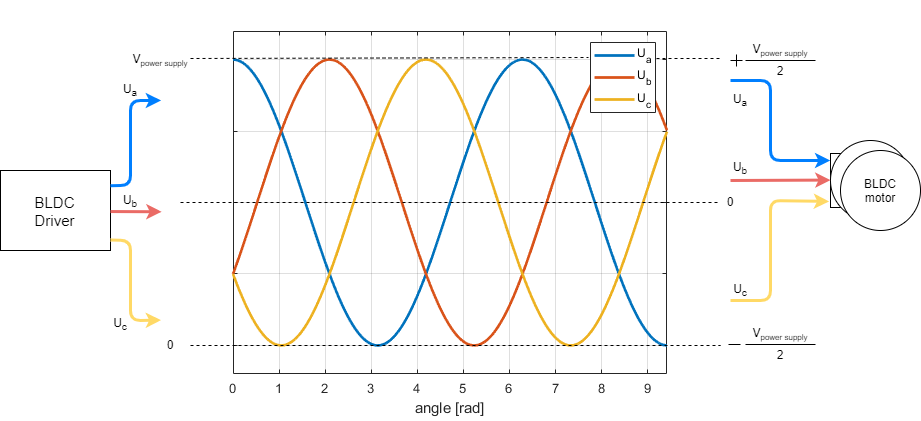### What happens when I exceed maximal Uq?

If you try to put voltage Uq higher than Uq = 0.5 Vpower_supply for SinePWM or Uq = 0.58 Vpower_supply for SpaceVectorPWM, it will still work but the Ua,b,c signals will be saturated.

Here are few images of the SinePWM for different Uq values.

Uq = 0.5 Vpower_supply Uq = 0.6 Vpower_supply Uq = Vpower_supply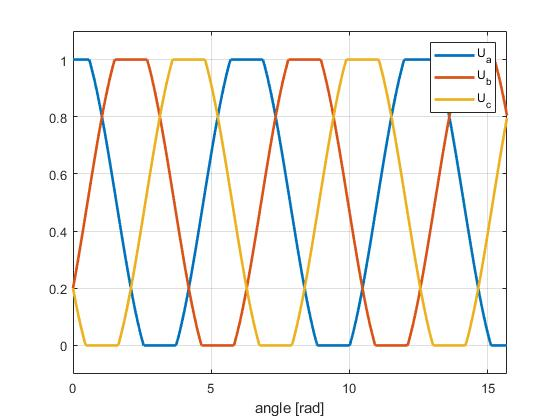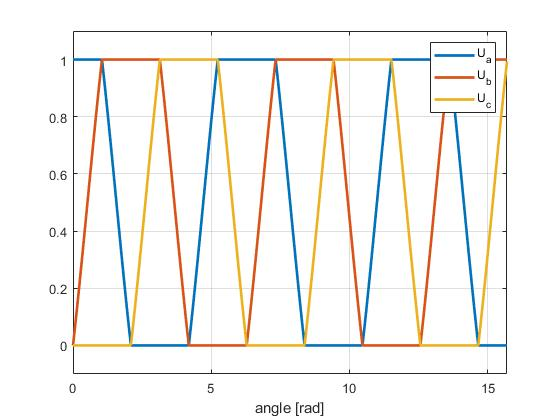Basically what you can see on images is that the Ua,b,c are saturated and you are actually not setting sinusoidal waves to your motor any more after you surpass the maximal values. The motor is still getting some increase of power, but it is no longer linear or smooth.

RULE OF THUMB

In reality the motor can see the difference all the way till Uq ~ 0.7 Vpower_supply . After this value the Ua,b,c are too saturated and further increase of Uq doesn't result in increase of motor power. But every motor is a bit different and you can check these values empirically on your own easily. Just put the motor in the voltage control and see after which value of the voltage Uq you can no longer see the improvement in motor power (it will stop accelerating).